# 2-3 Trees - Data Structures and Algorithms in C++

A 2-3 Tree is a type of tree in data structures in which every node of the tree is either a 2 node

or 3 nodes. It is a special type of B-Tree with order 3.

A 2 node in the tree is one which has one data part and two child nodes.

A 3 node in the tree is one which has two data parts and three child nodes.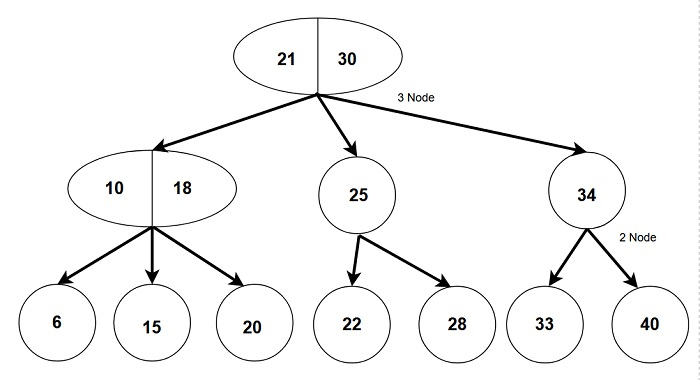## Properties of a 2-3 Tree:-

• Every internal node is either a 2 node or a 3 node.

• A node containing one data part can be a 2 node with exactly 2 children or a leaf node with no children.

• A node containing two data parts can only be a 3 node with exactly 3 children.

• All leaf nodes are always at the same level.

• A 2-3 tree is always a height balanced tree.

• Search operations are fast and efficient in a 2-3 tree.

## 2 Node:-

• Exactly two children.

• Left child with smaller weight.

• Right child with greater weight.

• Can be a leaf node with no child.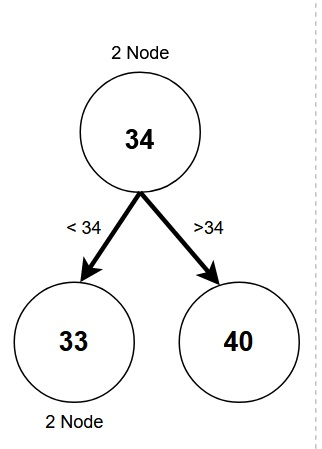## 3 Node:-

• Exactly three children.

• 2 data values.

• Left child with weight smaller than left data value.

• Middle child with weight in between both data values.

• Right child with weight greater than right data value.

• Can never be a leaf node.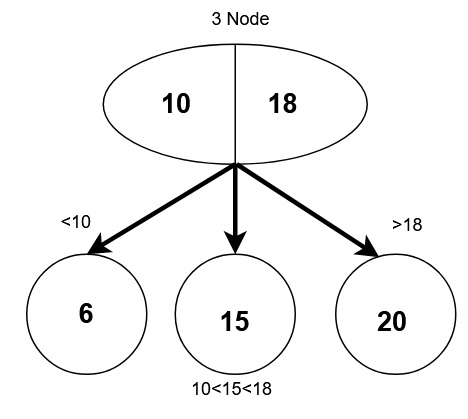## 1. Searching in a 2-3 tree

• Similar to the search operation in a binary search tree as the data is sorted.

• To search X in a 2-3 tree.

• If tree is empty → return False

• If X is less than the left data part, then search the left subtree

• If X is greater than left data and more than right data, search the middle subtree.

• If X is greater than the right data part, then search right subtree.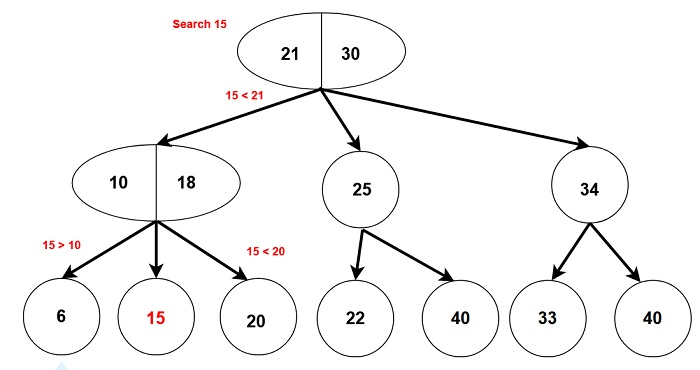## 2. Inserting a node in a 2-3 tree

• To insert X in a 2-3 tree.

• If the tree is empty → Add X as root.

• Search for the right position of X and add it as a leaf node.

• If there is a leaf node with one data part, add X with and leaf node becomes 2 - Node.

• If the leaf node has two data parts, add X. Split temporary 3-nodes and move data to parent nodes according to the sorting order.

Make 2-3 tree and add nodes in order → 10, 5, 8, 15, 23, 21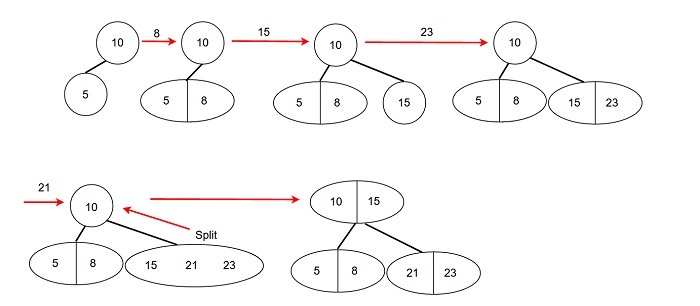## 3. Deletion of a node in a 2-3 tree

• To delete X in a 2-3 tree.

• If the tree is empty return false.

• Search for the position of X and delete it, then adjust the tree.

• If X is part of 3 node delete X and adjust left value and middle value. Also adjust node’s ancestor’s left and middle value if necessary.

• If X is part of 2 node then adjust and split the tree in a recursive manner arranging nodes in sorted order.